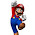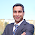Sunday, 13 June 2010

Broken axis, once more

I have discussed this subject at least on two occasions, and in fact, most of the present post was already described in one of my very first posts. However, I thought that it might be worthwhile to dust it off, especially, that this is a scientifically relevant feature, which is still missing in gnuplot. But with a bit of work, we can rectify the problem. In short, there are situations, when we just have to break the axis, simply because there is a large gap between two relevant ranges of a plot. Take this example, for instance
```plot [0:40] 20.0*atan(x-20.0) + 32 + sin(x)
```
which would result in the following graph:

The problem is obvious: the function has some interesting modulation close to 0, and close to 60, but it is rather dull between these two extrema. The solution is to cut out the segment between 10, and 60, say.

What we will use is a very handy function in gnuplot 4.4, which lets the user set the position of the graph exactly. In a multiplot, we would usually set the position as
```set multiplot
set size 1, 0.3
set origin 0, 0.5
plot 'foo' using 1:2

set size 1, 0.2
set origin 0, 0
plot 'bar' using 3:4
```
which produces two graphs of size 1, 0.3, and 1, 0.2, respectively, and places them in such a way that their bottom left corner is at (0, 0.5), and (0, 0). But when we say "the bottom left corner", we actually mean the whole figure, tic marks, axis labels, everything. This means at least two things. One is that if we want to break the axis using a multiplot, the ranges will not necessarily be proportional on the figure, simply because the size referred to the size of the whole graph, and not only to the plotting area. Second, if the size of the tic labels is different in the two graphs, they will no longer be aligned properly. This would happen, e.g., if we were to plot over the ranges [0:9], and [1000:10009]: the first range requires labels of width 1, while the second labels of width 5, therefore, the second graph would be narrower, and its left vertical axis shifted to the right, at least, as far as the plotting area is concerned.

In gnuplot 4.4, however, one can set the positions of the plots, and not the whole graph. This is achieved by issuing a command similar to this
```set lmargin at screen 0.1
```
which aligns the left vertical axis of the graph with the point that is at screen position 0.1 along the horizontal direction. There are three more margins, rmargin, tmargin, and bmargin, setting the right hand side, the top, and the bottom of the graph. Specifying the plot's corners explicitly removes the above-mentioned problem with the alignments.

Having said this, our script could read as follows
```reset
unset key
bm = 0.15
lm = 0.12
rm = 0.95
gap = 0.03
size = 0.75
y1 = 0.0; y2 = 11.5; y3 = 58.5; y4 = 64.0

set multiplot
set xlabel 'Time [ns]'
set border 1+2+8
set xtics nomirror
set ytics nomirror
set lmargin at screen lm
set rmargin at screen rm
set bmargin at screen bm
set tmargin at screen bm + size * (abs(y2-y1) / (abs(y2-y1) + abs(y4-y3) ) )

set yrange [y1:y2]
plot [0:40] 20.0*atan(x-20.0) + 32 + sin(x)

unset xtics
unset xlabel
set border 2+4+8
set bmargin at screen bm + size * (abs(y2-y1) / (abs(y2-y1) + abs(y4-y3) ) ) + gap
set tmargin at screen bm + size + gap
set yrange [y3:y4]

set label 'Power [mW]' at screen 0.03, bm + 0.5 * (size + gap) offset 0,-strlen("Power [mW]")/4.0 rotate by 90

set arrow from screen lm - gap / 4.0, bm + size * (abs(y2-y1) / (abs(y2-y1)+abs(y4-y3) ) ) - gap / 4.0 to screen \
lm + gap / 4.0, bm + size * (abs(y2-y1) / (abs(y2-y1) + abs(y4-y3) ) ) + gap / 4.0 nohead

set arrow from screen lm - gap / 4.0, bm + size * (abs(y2-y1) / (abs(y2-y1)+abs(y4-y3) ) ) - gap / 4.0  + gap to screen \
lm + gap / 4.0, bm + size * (abs(y2-y1) / (abs(y2-y1) + abs(y4-y3) ) ) + gap / 4.0 + gap nohead

set arrow from screen rm - gap / 4.0, bm + size * (abs(y2-y1) / (abs(y2-y1)+abs(y4-y3) ) ) - gap / 4.0 to screen \
rm + gap / 4.0, bm + size * (abs(y2-y1) / (abs(y2-y1) + abs(y4-y3) ) ) + gap / 4.0 nohead

set arrow from screen rm - gap / 4.0, bm + size * (abs(y2-y1) / (abs(y2-y1)+abs(y4-y3) ) ) - gap / 4.0  + gap to screen \
rm + gap / 4.0, bm + size * (abs(y2-y1) / (abs(y2-y1) + abs(y4-y3) ) ) + gap / 4.0 + gap nohead

plot [0:40] 20.0*atan(x-20.0) + 32 + sin(x)

unset multiplot
```

The first couple of lines specify how big a figure we want to have: bm, lm, and rm are the bottom, left, and right margins, respectively. We also define the size of the gap, which we will have between the two plots. y1 through y4 are the definitions of our plot ranges. In other words, the interval between y2, and y3 will be cut out of our figure.

In the multiplot environment, we set the axes (for the bottom figure on the bottom, left, and right, while for the top figure on the top, left, and right), the axis labels (the vertical label we have to set by hand, for otherwise it would be centred on the vertical axis of the bottom or top figure, but not on the whole), and set the positions of the figures. Note that the definition used for tmargin and bmargin makes sure that the two plotted intervals are proportional. Before plotting the second curve, we also set four small headless arrows, which are meant to represent the break in the axes. It can be left out, if not desired, or they can be replaced by two dashed vertical lines.

This method can also be used, if one wants to plot a single curve or data set, but with logarithmic axis on one interval, and linear on the other.

1.Thank you! Just what I wanted.

2.Very useful!

3.reset
unset key
bm = 0.15
lm = 0.12
rm = 0.95
gap = 0.03
size = 0.75
y1 = 0.0; y2 = 11.5; y3 = 58.5; y4 = 64.0
#kk = 0.7 #relative height of bottom plot
# or to keep proportional scale
kk = (abs(y2-y1) / (abs(y2-y1) + abs(y4-y3) ) )

set multiplot
set xlabel 'Time [ns]'
set border 1+2+8
set xtics nomirror
set ytics nomirror
set lmargin at screen lm
set rmargin at screen rm
set bmargin at screen bm
set tmargin at screen bm + size * kk

set yrange [y1:y2]
plot [0:40] 20.0*atan(x-20.0) + 32 + sin(x)

unset xtics
unset xlabel
set border 2+4+8
set bmargin at screen bm + size * kk + gap
set tmargin at screen bm + size + gap
set yrange [y3:y4]

set label 'Power [mW]' at screen 0.03, bm + 0.5 * (size + gap) offset 0,-strlen("Power [mW]")/4.0 rotate by 90

set arrow from screen lm - gap / 4.0, bm + size * kk - gap / 4.0 to screen \
lm + gap / 4.0, bm + size * kk + gap / 4.0 nohead

set arrow from screen lm - gap / 4.0, bm + size * kk - gap / 4.0 + gap to screen \
lm + gap / 4.0, bm + size * kk + gap / 4.0 + gap nohead
set arrow from screen rm - gap / 4.0, bm + size *kk - gap / 4.0 to screen \
rm + gap / 4.0, bm + size * kk + gap / 4.0 nohead

set arrow from screen rm - gap / 4.0, bm + size * kk - gap / 4.0 + gap to screen \
rm + gap / 4.0, bm + size * kk + gap / 4.0 + gap nohead

plot [0:40] 20.0*atan(x-20.0) + 32 + sin(x)

unset multiplot

4.This comment has been removed by the author.

5.Thank for blog post sharing the information.
Animation courses in Chandigarh

6.